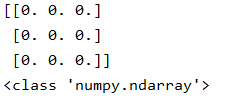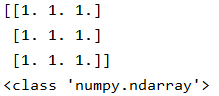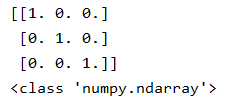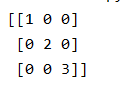• 一、自动生成全0二维数组 比如在实现逻辑回归和线性回归等算法时，需要先初始化一个权值矩阵，也就是要自动生成一个固定大小且各值全部相等的二维矩阵，这时候就可以用numpy来实现了。 下面的代码就是生成一个x行y...
（作者：陈玓玏）

一、自动生成全0二维数组

比如在实现逻辑回归和线性回归等算法时，需要先初始化一个权值矩阵，也就是要自动生成一个固定大小且各值全部相等的二维矩阵，这时候就可以用numpy来实现了。

下面的代码就是生成一个x行y列的全为0的矩阵。

import numpy as np
array = np.ones((x,y))*0

二、使用range生成整数等差数列

在进行for循环时，常常需要生成整数等差数列作为list的可迭代变量的索引，方便遍历这些变量，使用range就可以实现了。

下面两行代码是两种常用的方式，第一种是顺序遍历，从listA开始逐个遍历到listA[-1]，也就是listA的第一个到最后一个，这里之所以不用担心超出索引，是因为range生成的是一个左闭右开区间的等差数列。第二种方式是倒序遍历，这种方法不常用，注意步长要写成-1，不是1。

[listA[v] for v in range(len(listA))]
[listA[v] for v in range(len(listA)-1,0,-1)]

三、使用arange生成小数等差数列

range只能生成整数等差数列，如果使用range来生成小数等差数列，会报TypeError: ‘float’ object cannot be interpreted as an integer这个错误。arange函数是numpy包里的，使用时记得先引入numpy。

 import numpy as np
print(np.arange(0,1,0.1))


还是要注意区间左闭右开的问题，上面的方法生成的就是只包含0，不包含1的list。这个方法在绘制ks曲线时会用得到，因为ks曲线要在[0,1]区间内取划分正负样本的阈值，依次来计算ks曲线。
展开全文numpy 数据分析
• 一：全0数组 import numpy as np nd6=np.zeros([3,3])#全0数 print(nd6) print(type(nd6)) 实验结果： 二：全1数组 import numpy as np nd7=np.ones([3,3])#全1数 print(nd7) print(type(nd7)) 实验结果：...
一：全0数组

import numpy as np
nd6=np.zeros([3,3])#全0数
print(nd6)
print(type(nd6))


实验结果：二：全1数组

import numpy as np
nd7=np.ones([3,3])#全1数
print(nd7)
print(type(nd7))

实验结果：三：单位阵

import numpy as np
nd8=np.eye(3)#3阶单位阵
print(nd8)
print(type(nd8))

实验结果：四：对角阵

import numpy as np
print(np.diag([1,2,3]))#三阶对角矩阵

实验结果：展开全文Numpy TensorFlow
• 数组生成函数 函数名 描述 ...将输入数据(可以是列表、元组、数组以及其他序列)转换为ndarray，如不显式指明数据类型，将自动推断，默认复制所有的输入数据 ...根据所给形状和类型数据生成全0数组
数组生成函数

函数名
描述

array
将输入数据(可以是列表、元组、数组以及其他序列)转换为ndarray，如不显式指明数据类型，将自动推断，默认复制所有的输入数据

asarray
将输入转换为ndarray，但如果输入已经是ndarray则不再复制

arange
Python内建函数range的数组版，返回一个数组

ones
根据给定形状和数据类型生成全1数组

ones_like
根据所给数组生成一个形状一样的全1数组

zeros
根据所给形状和类型数据生成全0数组

zeros_like
根据所给数组生成全一个形状一样的全0数组

empty
根据给定形状生成一个没有初始化数值的空数组

empty_like
根据所给数组生成一个形状一样但没有初始化数值的空数组

full
根据给定的形状和数据类型生成指定数值的数组

full_like
根据所给的数组生成一个形状一样但内容是指定数值的数组

eye，identity
生成一个N×N特征矩阵（对角线位置都是1，其余位置是0）


展开全文numpy 数据分析
• #生成全0数组,默认生成浮点数 a=np.zeros(5) print(a) #生成全1数组,默认生成浮点数 a=np.ones(5) print(a) #生成全1数组,指定生成整数类型 a=np.ones(5,dtype="int") print(a) #fill方法将数组设为指定值 a
本文是视频Python数据分析三剑客 数学建模基础 numpy、pandas、matplotlib的学习笔记。
————————————————————————————————————————————————————
文章目录笔记产生数组数组属性数组索引和切片多维数组花式索引where函数类型转换数组排序求和最大值最小值均值标准差方差相关系数矩阵多维数组numpy内置函数第二次课作业（1）创建一个1到10的数组，然后逆序输出。（2）创建一个长度为20的全1数组，然后变成一个4×5的二维矩阵并转置。（3）创建一个3x3x3的随机数组。 (提示: np.random.random)（4）从1到10中随机选取10个数，构成一个长度为10的数组，并将其排序。获取其最大值最小值，求和，求方差。（5）从1到10中随机选取10个数，构成一个长度为10的数组，选出其中的奇数。（6）生成0到100，差为5的一个等差数列，然后将数据类型转化为整数。（7）从1到10中随机选取10个数，大于3和小于8的取负数。（8）在数组[1, 2, 3, 4, 5]中相邻两个数字中间插入1个0。（9）新建一个5乘5的随机二位数组，交换其中两行？比如交换第一二行。（10）把一个10*2的随机生成的笛卡尔坐标转换成极坐标。（11）创建一个长度为10并且除了第五个值为1其余的值为2的向量。（12）创建一个长度为10的随机向量，并求其累计和。（13）将数组中的所有奇数替换成-1。（14）构造两个4乘3的二维数组，按照3种方法进行连接？（15）获取数组 a 和 b 中的共同项（索引位置相同，值也相同）。a=np.array([1,2,3,2,3,4,3,4,5,6])，b=np.array([7,2,10,2,7,4,9,4,9,8])（16）从数组 a 中提取 5 和 10 之间的所有项。
NumPy 教程|菜鸟教程
个人理解Numpy实际上更类似c语言中传统意义上的数组，而不同于python自带的list，使用Numpy可以产生矩阵，进行很多统计方面的操作。
笔记
产生数组
import numpy as np

#从列表产生数组
L=[0,1,2,3]
a=np.array(L)
print(a)

#从列表传入
a=np.array([1,2,3,4])
print(a)

#生成全0数组,默认生成浮点数
a=np.zeros(5)
print(a)

#生成全1数组,默认生成浮点数
a=np.ones(5)
print(a)
#生成全1数组,指定生成整数类型
a=np.ones(5,dtype="int")
print(a)

#fill方法将数组设为指定值
a=np.array([1,2,3,4])
a.fill(5)
print(a)

#数组中元素的类型是一样的，而列表可以不同，若传入参数与数组类型不同会发生强制类型转换

#对a数组类型进行强制类型转换
a=a.astype('float')
a.fill(2.5)
print(a)

#生成整数序列
a=np.arange(1,10)

#生成等差序列(左闭右闭)
a=np.linspace(1,10,20)#20为元素个数
print(a)

#生成随机数
a=np.random.random(10)#10为元素个数
#或
a=np.random.rand(10)
print(a)

#生成服从标准正态分布的随机数
a=np.random.randn(10)
print(a)

#生成随机整数
a=np.random.randint(1,10,5)
print(a)

数组属性
#查看数组类型
type(a)

#查看数组数据类型
a.dtype

#查看形状，返回一个元组，每个元素代表这一维的元素数目
a.shape

#查看数组元素数目
a.size

#查看数组维度
a.ndim

数组索引和切片
#索引第一个元素
a

#修改第一个元素的值
a=10

#切片，支持负索引,左闭右开
a[1:3]
a[1:-1]

#省略参数
a[:1]
a[1:]
a[::2]#步长为2取数


多维数组
#生成多维数组
a=np.array([[1,2,3,4],[10,11,12,13]])

#查看形状
a.shape

#查看元素个数
a.size

#查看维度
a.ndim

#索引
a[1,3]
a   #第二行
a[:1]  #第二列

#切片
a=np.array([[0,1,2,3,4],
[10,11,12,13,14],
[20,21,22,23,24],
[30,31,32,33,34],
[40,41,42,43,44]])

#取第一行第四个和第五个元素
a[0,3:5]

#取最后两行的最后两列
a[3:,3:]

#取第三列
a[:,2]

#取3，5，行奇数列
a[2::2,::2]


数组中的切片是引用机制，列表不是
花式索引
可以实现任意位置的操作
#一维
a=np.arange(0,100,10)

#花式索引需要指定索引位置
index=[1,2,-3]
y=a[index]
print(y)

mask=np.array([0,1,1,1,0,0,0,1,1,0],dtype=bool)
print(a[mask])

#二维
b=np.array([[0,1,2,3,4],
[10,11,12,13,14],
[20,21,22,23,24],
[30,31,32,33,34],
[40,41,42,43,44]])

x=b[(0,1,2,3,4),(0,1,2,3,4)]
print(x)

#返回最后三行的第1，3，5列
print(b[2:,[0,2,4]])

#bool数组进行索引
mask=np.array([0,1,0,0,1],dtype=bool)
print(b[mask,2])


where函数
返回满足条件元素的索引位置，返回一个元组
#数组中大于10的元素的索引位置
a=np.array([0,11,52,2])
a=np.where(a>10)
print(a)
#数组操作
a[a>10]
a[np.where(a>10)]

类型转换
a=np.array([1,5,-3],dtype=float)

#asarray函数
np.asarray(a,dtype=int)

#astype函数,返回新数组
a.astype(int)

数组排序
#sort函数,数组本身顺序不变
np.sort(a)

#argsort函数，返回从小到大的排列在数组中的索引位置
order=np.argsort(a)

求和
np.sum(a)
a.sum()

最大值
np.max(a)
a.max()

最小值
np.min(a)
a.min()

均值
np.mean(a)
a.mean()

标准差
np.std(a)
a.std()

方差
np.var(a)
a.var()

相关系数矩阵
np.cov(a,b)

多维数组
#数组形状
a=np.arange(6)

a.shape=2,3

#reshape形成新数组，不修改原数组值
a.reshape(2,3)

#转置

a.T
a.transpose()

#数组连接
x=np.array([[0,1,2],[10,11,12]])
y=np.array([[5,1,2],[15,11,13]])
#默认沿第一维进行连接(纵向)
np.concatenate((x,y))
np.vstack((x,y))

#默认沿第二维进行连接(横向)
np.concatenate((x,y),axis=1)
np.hstack((x,y))

#连接成三维数组
z=np.array((x,y))
np.dstack((x,y))

numpy内置函数
内置函数总结
#求绝对值
np.abs(a)

#求指数幂
np.exp(a)

#求中值
np.median(a)

#求前缀和
np.cumsum(a)

第二次课作业
import numpy as np

（1）创建一个1到10的数组，然后逆序输出。
a=np.arange(1,11)
print(a[::-1])

[10  9  8  7  6  5  4  3  2  1]

（2）创建一个长度为20的全1数组，然后变成一个4×5的二维矩阵并转置。
a=np.ones(20,dtype='int')
a.shape=4,5
a.T

array([[1, 1, 1, 1],
[1, 1, 1, 1],
[1, 1, 1, 1],
[1, 1, 1, 1],
[1, 1, 1, 1]])

（3）创建一个3x3x3的随机数组。 (提示: np.random.random)
a=np.random.random([3,3,3])
a

array([[[0.11240525, 0.86131779, 0.69244856],
[0.6167795 , 0.09943477, 0.71824049],
[0.81575193, 0.45471067, 0.75354193]],

[[0.91707385, 0.02416993, 0.47008803],
[0.4392536 , 0.80667005, 0.1034436 ],
[0.69760784, 0.00658194, 0.68001535]],

[[0.34755532, 0.37713909, 0.74158849],
[0.52141301, 0.72062875, 0.88993301],
[0.78632516, 0.06687618, 0.79683884]]])

（4）从1到10中随机选取10个数，构成一个长度为10的数组，并将其排序。获取其最大值最小值，求和，求方差。
a=np.random.randint(1,10,10)
print(a)
a.sort()
print(a)
print(a.max())
print(a.min())
print(a.sum())
print(a.var())

[8 3 7 9 9 6 9 3 6 5]
[3 3 5 6 6 7 8 9 9 9]
9
3
65
4.85

（5）从1到10中随机选取10个数，构成一个长度为10的数组，选出其中的奇数。
a=np.random.randint(1,10,10)
for i in a:
if(i%2==1):
print(i)

5
1
3
9
9
7
7

（6）生成0到100，差为5的一个等差数列，然后将数据类型转化为整数。
a=np.linspace(0,100,21)
a=a.astype("int")
a

array([  0,   5,  10,  15,  20,  25,  30,  35,  40,  45,  50,  55,  60,
65,  70,  75,  80,  85,  90,  95, 100])

（7）从1到10中随机选取10个数，大于3和小于8的取负数。
a=np.random.randint(1,10,10)
for i in a:
if(a[i]>3 and a[i]<8):
a[i]=-a[i]
a

array([ 7,  6, -5,  2, -6, -4, -6, -4,  8,  6])

（8）在数组[1, 2, 3, 4, 5]中相邻两个数字中间插入1个0。
a=[1, 2, 3, 4, 5]
num=-1
for i in np.arange(0,len(a)-1):
num+=2
print(num)
a.insert(num,0)
a=np.array(a)
print(a)

1
3
5
7
[1 0 2 0 3 0 4 0 5]

a=[1, 2, 3, 4, 5]
b=np.zeros(9,dtype="int")
b[::2]=a
b

array([1, 0, 2, 0, 3, 0, 4, 0, 5])

（9）新建一个5乘5的随机二位数组，交换其中两行？比如交换第一二行。
a=np.random.random([5,5])
print(a)
b=a[]
a[]=a[]
a[]=b
a

a=np.random.random([5,5])
print(a)
a=a[[1,0,2,3,4]]

（10）把一个10*2的随机生成的笛卡尔坐标转换成极坐标。
a=np.random.randint(1,10,(10,2))
#print(a)
x=a[:,0]
y=a[:,1]
r=np.sqrt(x**2+y**2)
degree=np.arctan(y/x)
out=r,degree
print(out)

(array([10.63014581,  3.16227766, 10.81665383, 12.04159458,  8.06225775,
6.70820393,  7.28010989,  5.38516481,  5.38516481,  9.05538514]), array([0.85196633, 0.32175055, 0.5880026 , 0.72664234, 0.51914611,
0.46364761, 0.27829966, 1.19028995, 1.19028995, 1.46013911]))

（11）创建一个长度为10并且除了第五个值为1其余的值为2的向量。
a=(np.ones(10,dtype=int)+1)
a=1
a

array([2, 2, 2, 2, 1, 2, 2, 2, 2, 2])

（12）创建一个长度为10的随机向量，并求其累计和。
a=np.random.rand(10)
print(a.cumsum())

[0.61078914 1.44335701 1.54749383 2.40483    2.56192702 2.91247071
3.41405997 3.93025431 4.70034009 4.83646201]

（13）将数组中的所有奇数替换成-1。
a=np.random.randint(1,10,10)
a[a%2==1]=-1
a

array([ 2,  4,  4,  8,  6,  4, -1,  4, -1,  6])

（14）构造两个4乘3的二维数组，按照3种方法进行连接？
a=np.random.randint(1,10,(4,3))
b=np.random.randint(1,10,(4,3))
print(np.vstack((a,b)))
print(np.hstack((a,b)))
print(np.dstack((a,b)))

[[9 8 6]
[7 1 7]
[1 5 3]
[9 7 3]
[7 9 7]
[3 8 2]
[9 6 5]
[8 2 6]]
[[9 8 6 7 9 7]
[7 1 7 3 8 2]
[1 5 3 9 6 5]
[9 7 3 8 2 6]]
[[[9 7]
[8 9]
[6 7]]

[[7 3]
[1 8]
[7 2]]

[[1 9]
[5 6]
[3 5]]

[[9 8]
[7 2]
[3 6]]]

（15）获取数组 a 和 b 中的共同项（索引位置相同，值也相同）。a=np.array([1,2,3,2,3,4,3,4,5,6])，b=np.array([7,2,10,2,7,4,9,4,9,8])
a = np.array([1,2,3,2,3,4,3,4,5,6])
b = np.array([7,2,10,2,7,4,9,4,9,8])
a[a==b]

array([2, 2, 4, 4])

（16）从数组 a 中提取 5 和 10 之间的所有项。
a=np.array([7,2,10,2,7,4,9,4,9,8])
a=np.array([7,2,10,2,7,4,9,4,9,8])
a[(a>5)&(a<10)]

array([7, 7, 9, 9, 8])



展开全文• 1. 实现两个数组相加，在数据量特别大的时候 产生数组： （1）从列表产生数组：a=[0,1,2,3] a=np.array(1) a ...（3）生成全0数组 np.zeros(5) （4）生成全1的数组 np.ones(5,dtype=’b...
• 1、numpy的安装 pip install numpy 2、numpy生成数组 2.1、使用numpy生成一维数组 ...生成全0的标准数组 生成全1的标准数组 生成指定值的标准数组 #生成10个元素都是100的一维数组 2.3、生成二维数组 ...机器学习 numpy
• 本文主讲Python中Numpy数组的类型、全0全1数组生成、随机数组数组操作、矩阵的简单运算、矩阵的数学运算。尽管可以用python中list嵌套来模拟矩阵，但使用Numpy库更方便。定义数组>>> import numpy as np>>> m = ...
• 本文主讲Python中Numpy数组的类型、全0全1数组生成、随机数组数组操作、矩阵的简单运算、矩阵的数学运算。 尽管可以用python中list嵌套来模拟矩阵，但使用Numpy库更方便。 定义数组 >>> import ...
• 本文主讲Python中Numpy数组的类型、全0全1数组生成、随机数组数组操作、矩阵的简单运算、矩阵的数学运算。 尽管可以用python中list嵌套来模拟矩阵，但使用Numpy库更方便。  定义数组 >>> import numpy as np >...
• Python 数据分析（一） NumPy基础：数组与向量化计算 1.数组生成函数： arange:生成连续数字的数组（从0开始） In : np.arange(15) ...Out: array([ 0, 1,...zeros：一次性创造全0数组 In : np.zeros(10...数据分析
• 生成全0数组 n = 100 temp = [0 for _ in range(n)] 判断是否为空 temp = [] if temp: print('empty') if not temp: print('not empty') 模拟栈 temp = [] temp.append(num) # 压入栈 temp.pop() # 弹出栈 temp[-1...
• 导入numpy：import numpy as np 一、numpy常用函数 1.数组生成函数 ...np.zeros(shape)：生成shape维度大小的全0数组 np.zeros_like(a)：生成与a各维度大小一致的全0数组 np.ones(shape)：生...numpy
• 立体索引np.zeros：构造0的矩阵np.ones()：构造是1的矩阵np.empty()：构造空矩阵np.zeros_like()：生成与已知矩阵维度一样的0矩阵np.ones_like()：生成与已知矩阵维度一样的1矩阵np.identity()：...
• numpy 基本属性 n维数组；数值运算 ...全0数组：np.zeros((row,col)) 全1数组：np.ones((row,col)) 有序数列：np.arange(num); np.arange(start,end,by) 改变形状：array.reshape((row,col)) 生成线段
• 1.numpy import numpy as np a=np.array([[1,2,3] [2,3,4]]) #列表转n维数组，在后面可以设置数组类型 dtype=np.int 为整数类型 a.ndim #维度 ...np.empty(shape) #生成全无限接近0... np.arange(2,
• # zeros函数是生成指定维数的全0数组 # # 3、ones # # ones函数是用于生成一个全1的数组 # # 4.eye # # eye函数用户生成指定行数的单位矩阵 # # 5、.T # # .T作用于矩阵，用作球矩阵的转置 # # 6...
• （1）用0~19的数字生成(4,5)的数组命名为a,查看a的维度；查看a的轴的个数；查看a元素的总个数; （2）创建元素为1,2,3,4的(2,2)的数组 b，查看b中元素类型。 （3）创建一个1的（4,4）的数组c;创建一个内容随机的(3,...
• 是否大写文本_是否字母文本_标题化文本_首字母转大写文本_到小写文本_到大写文本_大小写字符到小写文本_大小写翻转文本_拼接文本_居中文本_左对齐文本_右对齐文本_右对齐0文本_删左边全部空格文本_删右边全部...
• 了解拉丁超立方原理建议去youtube搜视频，最多就5min，讲得很通俗易懂，看了很多文章长篇大论duck不必，搞懂后原来只是一个简单抽样规则而已。 自己写代码 ... result.fill(0)#赋初值为0 # result=[
• python运算符与ifelse逻辑语句第一天上午回顾第二天下午回顾1第二天回顾2.7习题解答2.11习题解答2.26作业KaliLinux简介3.4实现五边形面积计算3.7随机字符生成3.8实数误差与四舍五入3.18解答与特殊字符if简介与空格...
• 埃拉托色尼筛选法是用来生成质数的经典计算机编程算法，一般用来衡量计算机的速度。 我们知道，质数是能被自己和1整除的整数。 2，3，5，7，11都是质数。 那么算法是如何实现质数的识别呢？ 1.2我们可以简化理解为：...
• 本文主讲Python中Numpy数组的类型、全0全1数组生成、随机数组数组操作、矩阵的简单运算、矩阵的数学运算。 尽管可以用python中list嵌套来模拟矩阵，但使用Numpy库更方便。 定义数组 >>> import numpy ...numpy
• 列表常用操作 - 连接 / 复制(复制元素和复制数组) / 长度 / 排序 / 倒转 / 查找 生成列表 - 使用range创建数字列表 / 生成表达式 / 生成器 元组的使用 - 定义元组 / 使用元组中的值 / 修改元组变量 / 元组和列表转换...
• numpy 是python的科学计算包。 numpy里面的数组会将所有元素统一为同一类型的数据，只要数组中存在小数，整个数组的数据类型都是float，且输出的...numpy中可以直接生成一些特殊的数组，比如全0数组，空数组，连续元
• @Sofice 数据科学 Numpy 用于Python数值计算基础包 引用：import numpy as np ndarray多维数组对象 生成 ...将列表，元组，数组等转化为...全0 empty，empty_like 空数组 full，full_like 指定数值 eye, ide机器学习 numpy
• 若zs为None，则自动补为全0数组 color - 全部或每一个顶点的颜色。None表示使用cmap参数映射颜色 size - 顶点的大小，整型或浮点型。若为0，则表示不绘制点，只绘制线 width - 线宽，0.0~10.0之间的浮点数。若...# python生成全0数组python 订阅